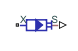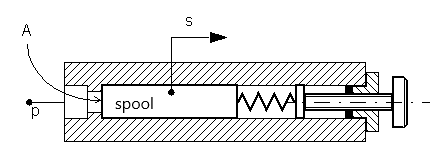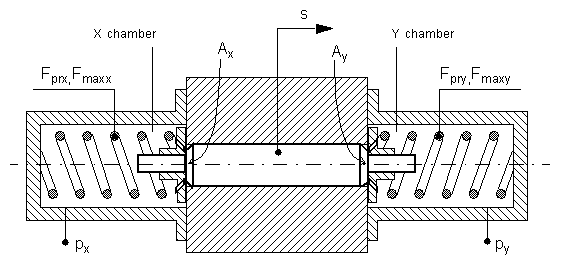# Pilot Valve Actuator (IL)

Single-acting or double-acting actuator controlled by a pilot pressure in an isothermal liquid network

Since R2020a

•Libraries:
Simscape / Fluids / Valve Actuators & Forces

## Description

The Pilot Valve Actuator (IL) block models a single-acting or double-acting actuator controlled by a pilot pressure for control of a connected valve or orifice in an isothermal liquid network.

For the single-acting actuator, when the control pressure, PXPatm, exceeds the Spring preload force at port X, the piston begins to actuate in the direction assigned by the Mechanical orientation parameter.

For the double-acting actuator, the control pressure Pcontrol is the difference between PXPatm and PYPatm. The piston actuates in the direction of the larger applied pressure differential, opposing the spring force at the opposite port. When the piston motion reverses, this spring does not extend and does not exert a counterbalancing force on the piston position.

The pistons at port X and port Y are attached to a single spool. Both springs restore the spool to its neutral position when Fspool falls below the opposing spring preload force. For single-acting actuators, the neutral position is at port X. For double-acting actuators, the neutral position is at the center of the actuator.

### Single-Acting Actuator

The force on the piston is created by the pressure differential between port X and atmospheric pressure:

`${F}_{spool}=\left({p}_{X}-{p}_{atm}\right){A}_{X},$`

where AX is the Piston area at port X. When Fspool is greater than the Spring preload force at port X, the piston begins to move.

Schematic of a Single-Acting ActuatorPiston Displacement

The instantaneous piston displacement is calculated as:

`${\stackrel{˙}{x}}_{dyn}=\frac{{x}_{steady}-{x}_{dyn}}{\tau },$`

where the steady piston position, xsteady, is the piston position at the current pressure differential, proportional to the spring force at maximum piston stroke:

`${x}_{steady}=F{x}_{stroke}\epsilon =\frac{{F}_{spool}-{F}_{pre}}{{F}_{\mathrm{max}}-{F}_{pre}}{x}_{stroke}\epsilon ,$`

where:

• Fpre is the Spring preload force at port X.

• Fmax is the maximum spring force acting against piston displacement, ${F}_{\mathrm{max}}=K{x}_{stroke}+{F}_{pre},$ and where K is the Spring stiffness at port X.

• xstroke is the Piston stroke from port X.

• ε is the Mechanical orientation, which indicates piston movement in a positive direction (extension) or negative direction (retraction).

If the force on the piston is less than the Spring preload force at X, the piston remains at the neutral position or moves to the neutral position. If the force on the piston meets or exceeds the maximum spring force, the piston remains at the stroke until the applied pressure changes.

### Double-Acting Actuator

The difference between the forces at ports X and Y dictates the piston motion:

`${F}_{spool}=\left({p}_{X}-{p}_{atm}\right){A}_{X}-\left({p}_{Y}-{p}_{atm}\right){A}_{Y}.$`

The pressure applied at port X shifts the spool away from the chamber at X and opposes the spring at port Y. Similarly, the pressure applied at port Y shifts the spool away from the chamber at Y and opposes the spring at port X. When the spool reverses direction, the formerly extended spring compresses, exerting a force on the spool. The formerly compressed spring, the spring at the port in the direction of motion, does not extend and does not influence the spool position.

Schematic of a Double-Acting ActuatorPiston Displacement

The piston displacement is calculated as:

`${\stackrel{˙}{x}}_{dyn}=\frac{{x}_{steady}-{x}_{dyn}}{\tau },$`

where the steady piston position, xsteady, is the piston position at the current pressure differential, proportional to the spring force at maximum piston stroke:

`${x}_{steady}=\left({F}_{Y}{x}_{stroke,Y}-{F}_{X}{x}_{stroke,X}\right)\epsilon =\left(\frac{{F}_{spool}-{F}_{pre,Y}}{{F}_{\mathrm{max},Y}-{F}_{pre,Y}}{x}_{stroke,Y}-\frac{\left(-{F}_{spool}-{F}_{pre,X}\right)}{{F}_{\mathrm{max},X}-{F}_{pre,X}}{x}_{stroke,X}\right)\epsilon ,$`

where:

• Fpre,X and Fpre,Y are the Spring preload force at port X and Spring preload force at port Y, respectively.

• Fmax,X and Fmax,Y are the maximum spring forces acting against piston displacement at ports X and Y, respectively, where:

`${F}_{\mathrm{max}}=K{x}_{stroke}+{F}_{pre},$`

K is the spring stiffness per port.

• xstroke is the piston stroke per port.

• ε is the Mechanical orientation, which assigns the signal for piston movement as positive (extension) or negative (retraction).

If the force on the piston is less than the respective port spring preload force, the piston remains or returns to the neutral position. If the force on the piston meets or exceeds the maximum spring force for the respective port, the piston remains at the piston stroke until the applied pressure changes.

### Numerically-Smoothed Force

When the actuator is close to full extension or full retraction, you can maintain numerical robustness in your simulation by adjusting the block . With a nonzero smoothing factor, a smoothing function is applied to all calculated forces, but primarily influences the simulation at the extremes of the piston motion.

When Actuator configuration is set to `Single-acting`, the normalized force on the piston is calculated as:

`${\stackrel{^}{F}}_{X}=\frac{{F}_{spool}-{F}_{Pre}}{{F}_{\mathrm{max}}-{F}_{Pre}}.$`

When Actuator configuration is set to `Double-acting`, the normalized force on the piston at X is calculated as:

`${\stackrel{^}{F}}_{X}=\frac{-{F}_{spool}-{F}_{Pre,X}}{{F}_{\mathrm{max},X}-{F}_{Pre,X}}.$`

and the normalized force at Y is calculated as:

`${\stackrel{^}{F}}_{Y}=\frac{{F}_{spool}-{F}_{Pre,Y}}{{F}_{\mathrm{max},Y}-{F}_{Pre,Y}}.$`

When the Smoothing factor is nonzero, the block smoothes each normalized force between `0` and `1`.

## Ports

### Conserving

expand all

Pressure at port X. There is no mass flow through the port.

Pressure at port Y. There is no mass flow through the port.

#### Dependencies

To enable this port, set Actuator type to `Double-acting`.

### Output

expand all

Piston displacement, specified as a physical signal. The displacement sign corresponds to the direction specified in the Mechanical orientation parameter.

## Parameters

expand all

Type of actuator modeled.

Cross-sectional area of the piston rod at port X.

Cross-sectional area of the piston rod at port Y.

#### Dependencies

To enable this parameter, set Actuator type to `Double-acting`.

Force acting against the piston in the neutral position.

#### Dependencies

To enable this parameter, set Actuator type to `Single-acting`.

Force at port X in the neutral position.

#### Dependencies

To enable this parameter, set Actuator type to `Double-acting`.

Force at port Y in the neutral position.

#### Dependencies

To enable this parameter, set Actuator type to `Double-acting`.

Spring stiffness factor. The maximum spring force is determined from the stiffness, K, and the Spring preload force: ${F}_{\mathrm{max}}=K{x}_{stroke}+{F}_{pre},$

#### Dependencies

To enable this parameter, set Actuator type to `Single-acting`.

Port X spring stiffness. The maximum spring force is determined from the stiffness, K, and the Spring preload force at port X: ${F}_{\mathrm{max}}=K{x}_{stroke}+{F}_{pre},$

#### Dependencies

To enable this parameter, set Actuator type to `Double-acting`.

Port Y spring stiffness. The maximum spring force is determined from the stiffness, K, and the Spring preload force at port Y: ${F}_{\mathrm{max}}=K{x}_{stroke}+{F}_{pre},$

#### Dependencies

To enable this parameter, set Actuator type to `Double-acting`.

Maximum piston travel distance.

#### Dependencies

To enable this parameter, set Actuator type to `Single-acting`.

Maximum piston travel distance at port X.

#### Dependencies

To enable this parameter, set Actuator type to `Double-acting`.

Maximum piston travel distance at port Y.

#### Dependencies

To enable this parameter, set Actuator type to `Double-acting`.

Continuous smoothing factor that introduces a layer of gradual change to the flow response when the valve is in near-open or near-closed positions. Set this value to a nonzero value less than one to increase the stability of your simulation in these regimes.

Whether to account for transient effects in the spool position due to actuation. Setting Actuator dynamics to `On` approximates actuator motion by introducing a first-order lag in the spool position. The Actuator time constant also impacts the modeled dynamics.

Constant that captures the time required for the piston to reach steady-state when moving from one position to another. This parameter impacts the modeled actuator dynamics.

#### Dependencies

To enable this parameter, set Actuator dynamics to `On`.

Sets the piston displacement direction based on the configuration at port X. If Actuator configuration is set to `Double-acting`, the displacement force at port Y acts in the opposite direction of the force at port X. ```Pilot pressure at port X causes positive piston displacement``` corresponds to positive displacement when the pressure at X pushes the piston towards port Y. ```Pilot pressure at port X causes negative piston displacement``` corresponds to negative displacement when the pressure at X pushes the piston towards port Y.

## Version History

Introduced in R2020a